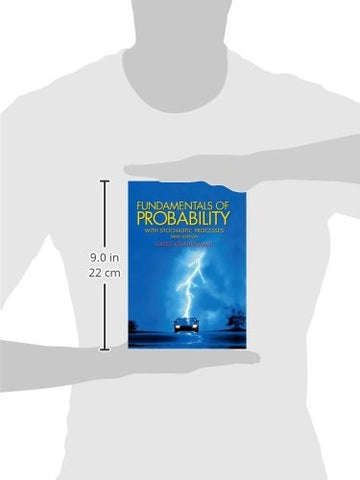# Fundamentals of Probability, with Stochastic Processes (3rd Edition)

• ISBN-13: 9780131453401
• \$87.35
• Save \$25

Only 3 left!

Presenting probability in a natural way, this book uses interesting, carefully selected instructive examples that explain the theory, definitions, theorems, and methodology. Fundamentals of Probability has been adopted by the American Actuarial Society as one of its main references for the mathematical foundations of actuarial science. Topics include: axioms of probability; combinatorial methods; conditional probability and independence; distribution functions and discrete random variables; special discrete distributions; continuous random variables; special continuous distributions; bivariate distributions; multivariate distributions; sums of independent random variables and limit theorems; stochastic processes; and simulation. For anyone employed in the actuarial division of insurance companies and banks, electrical engineers, financial consultants, and industrial engineers.